# GSEB Solutions Class 10 Maths Chapter 4 Quadratic Equations Ex 4.3

Gujarat Board GSEB Solutions Class 10 Maths Chapter 4 Quadratic Equations Ex 4.3 Textbook Questions and Answers.

## Gujarat Board Textbook Solutions Class 10 Maths Chapter 4 Quadratic Equations Ex 4.3

Question 1.±
Find the roots of the following quadratic equations, if they exist by the method of completing the square:Solution
2x2 – 7x + 3 = 0
x2 – $$\frac { 7 }{ 2 }$$x + $$\frac { 3 }{ 2 }$$ = 0
Adding and subtracting $$\left( \frac { 1 }{ 2 } \quad coefficient\quad of\quad x \right)$$2x = $$\frac { 12 }{ 4 }$$, $$\frac { 2 }{ 4 }$$ = 3, $$\frac { 1 }{ 2 }$$
Hence, the roots of the given quadratic equations are 3 and $$\frac { 1 }{ 2 }$$.

(ii)
2x2 + x – 4 = 0
x2 + $$\frac { 1 }{ 2 }$$x – 2 = 0
Adding and subtracting $$\left( \frac { 1 }{ 2 } \quad coefficient\quad of\quad x \right)$$2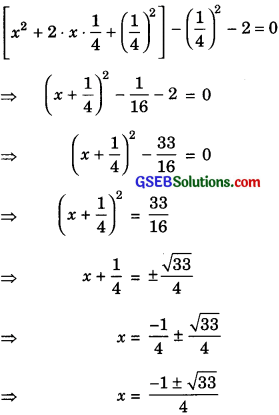Hence, the roots of the given quadratic equations are:
$$\frac { -1+\sqrt { 3 } }{ 4 }$$ and $$\frac { -1-\sqrt { 3 } }{ 4 }$$So, this root is repeated twice.
Hence, both the roots of the given equation are $$\frac { -\sqrt { 3 } }{ 2 }$$.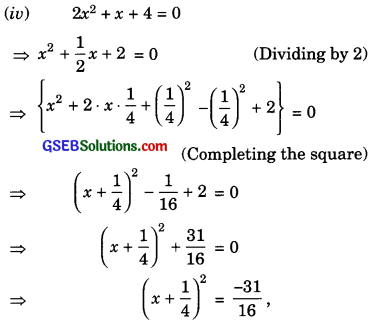But square of real numbers are not -ve.
Hence, the given quadratic equation has no real roots.Question 2.
Find the roots of the quadratic equations given in Q.1, above by applying the quadratic formula.
Solution:
(i)
We have,
2x2 – 7x + 3 = 0
Here, a = 2, b = – 7 and c = 3
Now, b2 – 4ac = (7)2 – 4 (2)(3)
= 49 – 24 = 25
Since, b2 – 4ac > 0
Therefore, the quadratic equation 2x2 – 7x + 3 = 0
has distinct roots i.e., α and β
Now,Therefore, the required roots are 3 and $$\frac { 1 }{ 2 }$$
(ii)
We have,
2x2 + x – 4 = 0
Here, a = 2, b = l and c = – 4
Now, b2 – 4ac = (1)2 – 4(2)(-4)
= 1 + 32 = 33
Since, b2 – 4ac > 0
Therefore, the quadratic equation 2x2 + x – 4 = 0 has distinct roots i.e., α and β
Now,(iii)
Wehave,
4x2 + 4$$\sqrt { 3 }$$x + 3 = 0
Here, a = 4, b = 4$$\sqrt { 3 }$$ and c = 3
Now, b2 – 4ac = (4$$\sqrt { 3 }$$)2 – 4(4) (3)
= 48 – 48 = 0
Since, b2 – 4ac = 0
4x2 + 4$$\sqrt { 3 }$$ + 3 = 0 has equal roots i.e., α = βTherefore, the required roots are $$\frac { -\sqrt { 3 } }{ 2 }$$

(iv)
We have,
2x2 + x + 4 = 0.
Here, a = 2, b = 1 and c = 4
Now, b2 – 4ac =(1)2 – 4(2)(4)
= 1 – 32 = – 31
Since, b2 – 4ac < 0
Therefore, the quadratic equation 2x2 + x + 4 = 0 has no real roots.Question 3.
Find the roots of the gollowing equations:solution:
(i) we have,
x – $$\frac { 1 }{ x }$$ = 3
$$\frac { { x }^{ 2 }-1 }{ x }$$ = 3
x2 – 1 = 3x
x2 – 3x – 1 = 0
Now, a = 1, b = – 3 and c = – 1
Now, b2 – 4ac = (-3)2 – 4(1)( – 1)
= 9 + 4 = 13
Sicne b2 – 4ac > 0
Therefore, the quadratic equation x2 – 3x – 1 = 0
has distinct roots i.e., a and 3.Therefore, the required roots are $$\frac { 3+\sqrt { 3 } }{ 2 }$$ and $$\frac { 3-\sqrt { 3 } }{ 2 }$$

(ii)
We have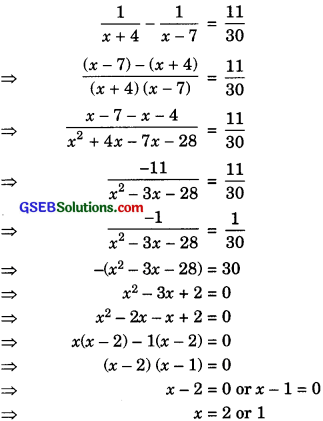So the roots of the equation are 2 and 1.Question 4.
The sum of the reciprocals of Rehman’s age (in years) 3 years ago and 5 years from now is Find his present age.
Solution:
Let the present age of Rehman be x years.
3 years ago, his age was (x – 3) years and
5 years from now his age will be (x +5) years.
Now, according to the given problem, we have
$$\frac { 1 }{ x-3 }$$ + $$\frac { 1 }{ x+5 }$$ = $$\frac { 1 }{ 3 }$$
$$\frac { x+5+x-3 }{ \left( x-3 \right) \left( x+5 \right) }$$ = $$\frac { 1 }{ 3 }$$
$$\frac { 2x+2 }{ { x }^{ 2 }+2x-15 }$$ = $$\frac { 1 }{ 3 }$$
x2 + 2x – 15 = 3(2x + 2)
x2 + 2x – 15 = 6x +6
x2 + 2x – 6x – 15 – 6 = 0
x2 – 4x – 21 = 0
x2 – 7x + 3x – 21 = 0
x (x – 7) + 3(x – 7) = 0
(x – 7)(x + 3) = 0
x – 7 = 0 or x + 3 = 0
x = 7 or x = – 3
But age cannot be -ve.
x = 7.
Hence, the present age of Rehman = 7 years.Question 5.
In a class, the sum of Shefali’s marks in Mathematics and English is 30. Had she got 2 marks more in Mathematics and 3 marks less in English, the product would have been 210. Find her marks in the two subjects.
Solution:
Let marks got by Shefali in Mathematics = x
Then, marks got by her in English = (30 – x)
Now, according to the given problem, we have
(x+ 2)(30 – x – 3) = 210
(x + 2)(27 – x) = 210
27x – x2 + 54 – 2x – 210 = 0
– x2 + 25 x -156 = 0
x2 – 25 x + 156 = 0
x2 – 13x – 12x + 156 = 0
x(x – 13) – 12(x – 13) = 0
(x – 13)(x – 12) = 0
x – 13 = 0 or x – 12 = 0
x = 13 or x = 12
Hence, if marks got by Shefali in Mathematics 13
then marks got by her in English 30 – 13 = 17
and if marks got by Shefali in Mathematics = 12,
then marks got by her in English = 30 – 12 = 18.Question 6.
The diagonal of a rectangular field is 60 metres more than the shorter side. If the longer side is 30 metres more than the shorter side, find the sides of the field.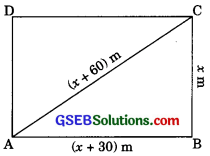Solution:
Let the shorter side of the field = x m
Then, longer side of the field = (x + 30) m and
diagonal of the field = (x + 60) m
Now, in right MBC, by Pythagoras theorem,
We have
AB2+ BC2 = AC2
(x + 30)2 + x2 = (x + 60)2
x2 + 60 x +900 + x2 = x2 + 120x + 3600
2x2 + 60x + 900 = x2 + 120x + 3600
2x2 + 60x – 120x + 900 – 3600 = 0
x2 – 60x – 2700 = 0
which is a quadratic equation in X.
We can solve it by factorization method
x2 – 60x – 2700 = 0
x2 – 90x + 30x – 2700 = 0
x(x – 90) + 30(x – 90) = 0
(x + 30) + (x – 90) = 0
x + 30 = 0 or x – 90 = 0
x = -30 or x = 90
But x = – 30 is not possible because aide cannot be negative.
x = 90
So, breadth of the rectangle = 90 m and length
of the rectangle = 90 + 30 = 120 m.Question 7.
A train travels 360 km at a uniform speed. If the speed had been 5 km/h more, would have taken 1 hour less for the sanie journey. Find the speed of the train.
Solution:
Let the speed of the train = x km/h
Distance = 360 km
Time taken to cover 360 km
If the speed had been 5 km/h more, then time $$\frac { 360 }{ x }$$h.
taken to cover 360 km = $$\frac { 360 }{ x }$$ h
Now, according to the given problem,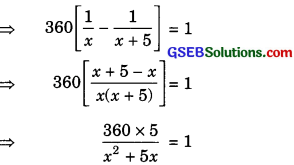x2 + 5x = 1800
x2 + 5x – 1800 = 0
which is a quadratic equation in x. It can be solved by factorization method.
x2 + 45x – 40x – 1800 = 0
x (x + 45) – 40(x + 45) = 0
– (x + 45)(x – 40) = 0
x + 45 = 0 or x – 40 = 0
x = – 45 or x = 40
But speed of the train cannot be -ve.
x = 40
Hence, speed of the train = 40 km/h.Question 8.
Two water taps together can fill a tank in hours. The larger tap takes 10 hours less than the smaller one to fill the tank separately. Find the time in which each tap can separately fill the tank.
Solution:
Let the time taken by the larger tap to fill the tank is x hours.
Time taken by the smaller tap to fill the tank =(x + 10) hours
Now, portion of the tank filled by the larger tank in 1 hour = $$\frac { 1 }{ x }$$
Portion of the tank filled by the smaller tank in
1 hour = $$\frac { 1 }{ x + 10 }$$ and portion of the tank filled
by the both in 1 hour
= $$\frac { 1 }{ x }$$ + $$\frac { 1 }{ x+10 }$$ = $$\frac { x }{ y }$$
$$\frac { 2x+10 }{ x\left( x+10 \right) }$$ = $$\frac { 2\left( x+5 \right) }{ x\left( x+10 \right) }$$ ……………. (1)
It is given that both taps together can fill in 9 $$\frac { 3 }{ 8 }$$h
so, portion of the tank filled by both in 1 hour = $$\frac { 8 }{ 75 }$$ ………..(2)
From (1) and (2), we get
$$\frac { 2\left( x+5 \right) }{ x\left( x+10 \right) }$$ = $$\frac { 8 }{ 75 }$$
$$\frac { x+5 }{ { x }^{ 2 }+10x }$$ = $$\frac { 4 }{ 75 }$$
75x + 375 = 4x2 + 40x
4x2 + 40x – 75x – 375 = 0
4x2 – 35x – 375 = 0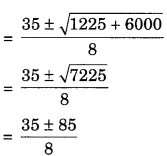=$$\frac { 35+85 }{ 8 }$$ or $$\frac { 35-85 }{ 8 }$$
= $$\frac { 120 }{ 8 }$$ or $$\frac { -50 }{ 8 }$$
= 15 or $$\frac { -50 }{ 8 }$$, but it is rejected.
Hence, time taken by the larger tap = 15 hours
and time taken by the smaller tap = (15 + 10)
= 25 hours.Question 9.
An express train takes 1 hour less than a passenger train to travel 132 km between Mysore and Bangalore (without taking
into consideration the time they stop at intermediate stations). If the average speed of the express train is 11 km/h more than that of the passenger train, find the average speed of the two trains.
Solution:
Let the average speed of the passenger train = x km/h
Then, the average speed of the express train = (x + 11)km/h
Distance = 132 km
Time taken by the passenger train to cover 132
= $$\frac { 132 }{ x }$$ h and the time taken by express train to
cover 132 km = $$\frac { 132 }{ x+11 }$$ h
Now, according to the given problem,
$$\frac { 132 }{ x }$$ – $$\frac { 132 }{ x+11 }$$ = 1
132$$\left[ \frac { 1 }{ x } +\frac { 1 }{ x+11 } \right]$$ = 1
$$\frac { x+11-x }{ x\left( x+11 \right) }$$ = $$\frac { 1 }{ 132 }$$
$$\frac { 11 }{ { x }^{ 2 }+11x }$$ = $$\frac { 1 }{ 132 }$$
x2 + 11x = 132 x 11
x2 + 11x – 1452 = 0
which is a quadratic equation in x.
We can solve this equation by quadratic
formula.
Here, a = 1, b = 11, c = – 1452
D = b2 – 4ac
(11)2 – 4 x 1 x 1(-1452)
= 121 + 5808 = 5929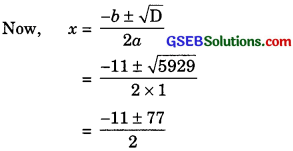$$\frac { 66 }{ 2 }$$, $$\frac { -88 }{ 2 }$$ = 33, -44
But speed of the train cannot be – ve.
x = 33
Hence, the average speed of the passengers
train = 33 km/h and the average speed of
express train = 33 + 11 = 44 km/h.Question 10.
Sum of the area of the two squares is 468 m2. If the difference of their perimeters is 24 m, find the sides of the two squares.
Solution:
Let the side of first square = x m
and the side of second square = y m
Then, the area of first square = x2 m2
and the area of second square = y2 m2
Perimeter of the first square = 4x m
and perimeter of the second square = 4y m
x2 + y2 = 468 ……………. (1)
and 4x – 4y = 24
x – y = 6 ……………(2)
From equation (2), we get
x = 6 + y …………..(3)
Putting this value of x in (1), we get
(6 + y)2 + y2 = 468
36 + 12y + y2 + y2 = 468
2y2 + 12y + 36 – 468 = 0
= 2y 2 + 12y – 432 = 0
y2 + 6y – 216 = 0
which is a quadratic equation in y.
We can solve this equation by quadratic formula.
Here,
a = 1, b = 6, c = – 216
D = b2 – 4ac
= (6)2 – 4 x 1 x ( – 216)
= 36 + 864 = 900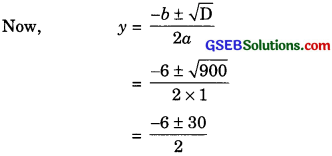But the side of a square cannot be – ve.
y = 12
Putting this value ofy in (3), we get
x = 6 + 12 = 18
Hence, the side of the first square = 18 m
and the side of the second square = 12 m.